# Check if a subtree exists with the given sum

In this article, we are going to see how we can check whether a subtree exists with the given sum or not?
Submitted by Radib Kar, on September 15, 2020

In this article, we are going to see how we can check if there is any subtree exists with the given sum. For example,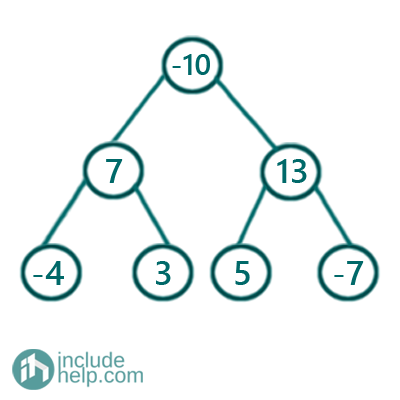There is a subtree if the given sum is 6 and the subtree is,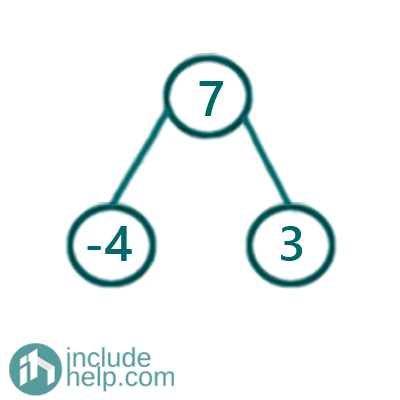Of course, the brute force method is to find subtree sum rooted by each node and to check if the subtree sum matches with the input sum. But the complexity would be O(n^2).

So, the efficient approach is a recursive solution using postorder traversal which will solve in one pass. The algorithm is below:

Initially have a flag variable, answer set to FALSE which will change if we find any subtree with the given sum. And input sum is X.

```FUNCTION maxSubtreeSumRecur(node, answer, X)
If node is NULL
return 0;
End IF
then we already found the subtree so simply return any number
End IF
current sum= node value + left subtree sum(find recursively) + right subtree sum (find recursively)
if current sum ==X
return current sum which is the subtree sum rooted by node;
END FUNCTION
```

So, it's a postorder traversal which returns the subtree sum and we need to check if subtree sum is the same as the given sum.

```#include <bits/stdc++.h>
using namespace std;

// tree node is defined
class TreeNode {
public:
int val;
TreeNode* left;
TreeNode* right;
TreeNode(int data)
{
val = data;
left = NULL;
right = NULL;
}
};

int maxSubtreeSumRecur(TreeNode* node, int X, bool& answer)
{
//if node is NULL return 0
if (!node)
return 0;
//if answer is already true, then return anything, no need to proceed
return INT_MIN;

int cur_sum = node->val + maxSubtreeSumRecur(node->left, X, answer) + maxSubtreeSumRecur(node->right, X, answer);

if (cur_sum == X)
return cur_sum;
}

bool maxSubtreeSum(TreeNode* root, int X)
{
if (!root)
return false;
}

int main()
{
//building the tree like the example
TreeNode* root = new TreeNode(-10);

root->left = new TreeNode(7);
root->right = new TreeNode(13);
root->left->left = new TreeNode(-4);
root->left->right = new TreeNode(3);
root->right->left = new TreeNode(5);
root->right->right = new TreeNode(-7);

int X = 6;

if (maxSubtreeSum(root, X))
cout << "There is subtree found with given sum " << X << endl;
else
cout << "There is no subtree found with given sum " << X << endl;

X = 12;
if (maxSubtreeSum(root, X))
cout << "There is subtree found with given sum " << X << endl;
else
cout << "There is no subtree found with given sum " << X << endl;

return 0;
}
```

Output:

```There is subtree found with given sum 6
There is no subtree found with given sum 12
```

Dry Run:

The tree is the given one same as example and the input sum is 6.

Since it's a postorder traversal we will show the dry run in bottom-up fashion as the recursion will reach their first before returning something.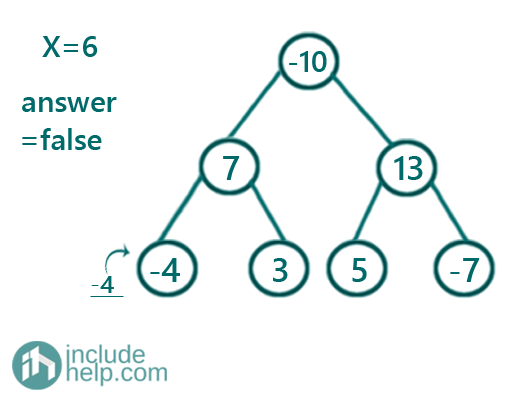Figure 1: cur_sum is 4 which is not equal to given sum 6, so answer is false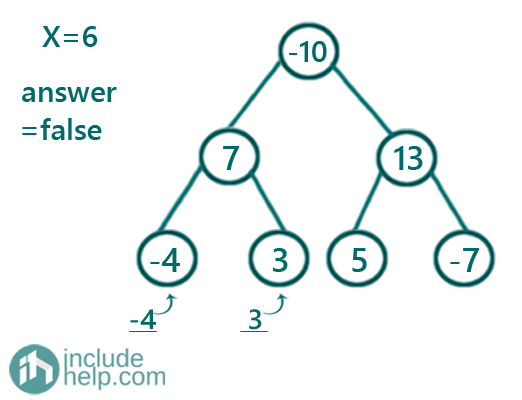Figure 2: cur_sum is 3 which is not equal to given sum 6, so answer is false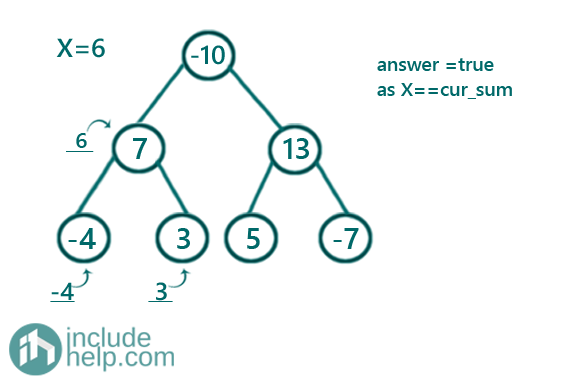Figure 3: cur_sum is 6 which is not equal to given sum 6, so answer is true

Since the answer is true now in each further recursion it will simply return and reach the main calling function with answer = true. So, there is a subtree with the given sum 6.

What's New

Top Interview Coding Problems/Challenges!

Languages: » C » C++ » C++ STL » Java » Data Structure » C#.Net » Android » Kotlin » SQL
Web Technologies: » PHP » Python » JavaScript » CSS » Ajax » Node.js » Web programming/HTML
Solved programs: » C » C++ » DS » Java » C#
Aptitude que. & ans.: » C » C++ » Java » DBMS
Interview que. & ans.: » C » Embedded C » Java » SEO » HR
CS Subjects: » CS Basics » O.S. » Networks » DBMS » Embedded Systems » Cloud Computing
» Machine learning » CS Organizations » Linux » DOS
More: » Articles » Puzzles » News/Updates